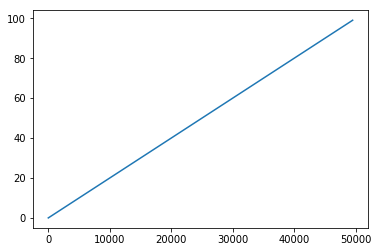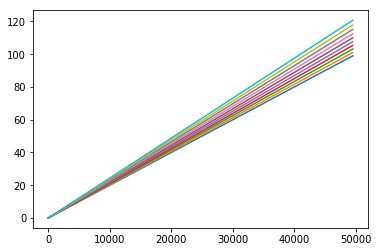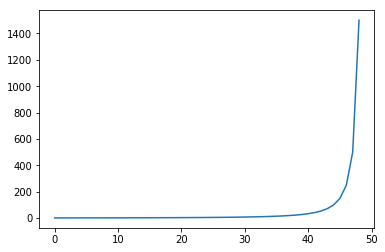First, let's fix Python 2 division gotcha (will do nothing on Python 3).

In :
from __future__ import division


Now let's calculate some growth on the path. Let's say we have a path of 50km or 50000m length.

In :
pathlen = 50000  # meters


Now imagine that we have something growing every 500 meters by 1 meter.

In :
growthstep = 500
growthrate = 1/growthstep


So at 1000 meters we'll get something at 2 meters high

In :
growthrate * 1000

Out:
2.0

аnd at 50000m mark..

In :
growthrate * pathlen

Out:
100.0

Let's see how it looks with pyplot.

In :
from matplotlib import pyplot

In :
plt = pyplot
#plt.plot(pathlen)
plt.show()


It needs some x,y points to draw.

In :
xy = [[step, step*growthrate] for step in range(0, pathlen, 500)]
x,y = zip(*xy)

In :
plt.plot(x, y)
plt.show()Using arrays from numpy is easier

In :
import numpy as np

In :
# create a range from 0 to 50000 with 500 step
r = np.arange(0, 50000, 500)

In :
plt.plot(r, r*growthrate)
plt.show()Now let's shorten the growth cycle in 10 meters interval

In :
for x in range(0, 10):
rate = 1/(growthstep-10*x)
plt.plot(r, r*rate)

In :
plt.show()Let's measure the height at mark 30000 at different growth rates.

In :
growth = []
for x in range(0, 50):
rate = 1/(growthstep-10*x)
pair = [growthstep-10*x, 30000*rate]
growth.append(pair)
print pair

[500, 60.0]
[490, 61.22448979591837]
[480, 62.5]
[470, 63.829787234042556]
[460, 65.21739130434783]
[450, 66.66666666666667]
[440, 68.18181818181817]
[430, 69.76744186046511]
[420, 71.42857142857143]
[410, 73.17073170731707]
[400, 75.0]
[390, 76.92307692307692]
[380, 78.94736842105263]
[370, 81.08108108108108]
[360, 83.33333333333334]
[350, 85.71428571428571]
[340, 88.23529411764706]
[330, 90.9090909090909]
[320, 93.75]
[310, 96.7741935483871]
[300, 100.0]
[290, 103.44827586206897]
[280, 107.14285714285714]
[270, 111.11111111111111]
[260, 115.38461538461539]
[250, 120.0]
[240, 125.0]
[230, 130.43478260869566]
[220, 136.36363636363635]
[210, 142.85714285714286]
[200, 150.0]
[190, 157.89473684210526]
[180, 166.66666666666669]
[170, 176.47058823529412]
[160, 187.5]
[150, 200.0]
[140, 214.28571428571428]
[130, 230.76923076923077]
[120, 250.0]
[110, 272.7272727272727]
[100, 300.0]
[90, 333.33333333333337]
[80, 375.0]
[70, 428.57142857142856]
[60, 500.0]
[50, 600.0]
[40, 750.0]
[30, 1000.0]
[20, 1500.0]
[10, 3000.0]


And calculate height difference for every 10 decrement in growth range

In :
delta = []
for i in range(len(growth)-1):
delta.append(growth[i+1]-growth[i])
print delta[-1]

1.22448979592
1.27551020408
1.32978723404
1.38760407031
1.44927536232
1.51515151515
1.58562367865
1.66112956811
1.74216027875
1.82926829268
1.92307692308
2.02429149798
2.13371266003
2.25225225225
2.38095238095
2.52100840336
2.67379679144
2.84090909091
3.02419354839
3.22580645161
3.44827586207
3.69458128079
3.96825396825
4.2735042735
4.61538461538
5.0
5.4347826087
5.92885375494
6.49350649351
7.14285714286
7.89473684211
8.77192982456
9.80392156863
11.0294117647
12.5
14.2857142857
16.4835164835
19.2307692308
22.7272727273
27.2727272727
33.3333333333
41.6666666667
53.5714285714
71.4285714286
100.0
150.0
250.0
500.0
1500.0

In :
plt.plot(delta)
plt.show()In [ ]:


In [ ]: